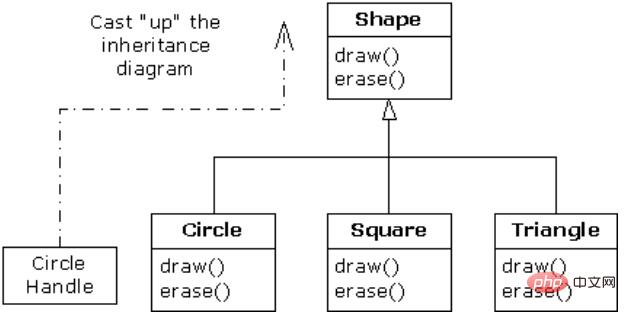# Java中继承图文详解1.继承的语法

```class Student extends Person {

}```

2.合成的语法

```class Student {
Dog dog;
}```

1.基本概念

```class Person {
public void eat() {
System.out.println("eat");
}

static void show(Person p) {
p.eat();
}
}
public class Student extends Person{
public static void main(String[] args) {
Student s = new Student();
Person.show(s);     // ①
}
}```

【运行结果】：
eat

2.为什么要上溯造型

```class Value {
private int count = 1;

private Value(int count) {
this.count = count;
}

public static final Value
v1 = new Value(1),
v2 = new Value(2),
v3 = new Value(3);
}

class Person {

public void eat(Value v) {
System.out.println("Person.eat()");
}
}

class Teacher extends Person {
public void eat(Value v) {
System.out.println("Teacher.eat()");
}
}

class Student extends Person {
public void eat(Value v) {
System.out.println("Student.eat()");
}
}

public class UpcastingDemo {
public static void show(Student s) {
s.eat(Value.v1);
}

public static void show(Teacher t) {
t.eat(Value.v1);
}

public static void show(Person p) {
p.eat(Value.v1);
}

public static void main(String[] args) {
Student s = new Student();
Teacher t = new Teacher();
Person p = new Person();
show(s);
show(t);
show(p);
}
}```

```public static void show(Person p) {
p.eat(Value.v1);
}```

```public static void show(Person p) {
p.eat(Value.v1);
}```

1.方法调用的绑定`Shape s = new Shape();`

`Shape s = new Shape();`

```class Person {
void eat() {}
void speak() {}
}
class Boy extends Person {
void eat() {
System.out.println("Boy.eat()");
}
void speak() {
System.out.println("Boy.speak()");
}
}
class Girl extends Person {
void eat() {
System.out.println("Girl.eat()");
}
void speak() {
System.out.println("Girl.speak()");
}
}
public class Persons {
public static Person randPerson() {
switch ((int)(Math.random() * 2)) {
default:
case 0:
return new Boy();
case 1:
return new Girl();
}
}
public static void main(String[] args) {
Person[] p = new Person;
for (int i = 0; i < p.length; i++) {
p[i] = randPerson();    // 随机生成Boy或Girl
}
for (int i = 0; i < p.length; i++) {
p[i].eat();
}
}
}```

```class Person {
void eat() {
System.out.println("Person.eat()");
}
}
class Boy extends Person {
void eat() {
System.out.println("Boy.eat()");
}
void speak() {
System.out.println("Boy.speak()");
}
}
public class Persons {
public static void main(String[] args) {
Person p = new Boy();
p.eat();
p.speak();  // The method speak() is undefined for the type Person
}
}```

```class Person {
void eat() {
System.out.println("Person.eat()");
}
}
class Boy extends Person {
}
public class Persons {
public static void main(String[] args) {
Person p = new Boy();
p.eat();
}
}```

【运行结果】:
Person.eat()

2.静态方法的绑定

```class Person {
static void eat() {
System.out.println("Person.eat()");
}
static void speak() {
System.out.println("Person.speak()");
}
}
class Boy extends Person {
static void eat() {
System.out.println("Boy.eat()");
}
static void speak() {
System.out.println("Boy.speak()");
}
}
class Girl extends Person {
static void eat() {
System.out.println("Girl.eat()");
}
static void speak() {
System.out.println("Girl.speak()");
}
}
public class Persons {
public static Person randPerson() {
switch ((int)(Math.random() * 2)) {
default:
case 0:
return new Boy();
case 1:
return new Girl();
}
}
public static void main(String[] args) {
Person[] p = new Person;
for (int i = 0; i < p.length; i++) {
p[i] = randPerson();    // 随机生成Boy或Girl
}
for (int i = 0; i < p.length; i++) {
p[i].eat();
}
}
}```

【运行结果】：

```Person.eat()
Person.eat()
Person.eat()
Person.eat()```63K